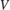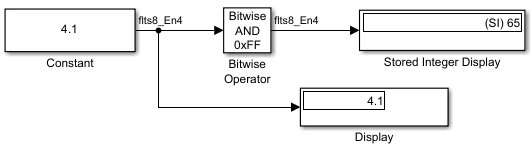# Use Scaled Doubles to Avoid Precision Loss

This example shows how you can avoid precision loss by overriding the data types in your model with scaled doubles.

### Open the Model

Open the `ex_scaled_double` model.

```ex_scaled_double ```In the `ex_scaled_double` model, the Constant block Output data type is `fixdt(1,8,4)`. The Bitwise Operator block uses the `AND` operator and the bit mask `0xFF` to pass the input value to the output. Because the Treat mask as parameter is set to `Stored Integer`, the block outputs the stored integer valueof its input. The encoding scheme is, whereis the real-world value andis the stored integer value.

### Collect Ranges in the Fixed-Point Tool

1. From the Simulink® Apps tab, select Fixed-Point Tool.

2. Select the Range Collection workflow.

3. In the Fixed-Point Tool, select Collect Ranges > Use current settings. Click Collect Ranges.The Display block displays `4.125` as the output value of the Constant block. The Stored Integer Display block displays `(SI) bin 0100 0010`, which is the binary equivalent of the stored integer value. Precision loss occurs because the output data type, `fixdt(1,8,4)`, cannot represent the output value `4.1` exactly.

### Override Data Types with Scaled Doubles

Override data types in the model with scaled doubles.

1. In the Fixed-Point Tool, select New > Range Collection to start a new workflow.

2. Select Collect Ranges > Scaled double precision. Click Collect Ranges.The Display block correctly displays `4.1` as the output value of the Constant block. The Stored Integer Display block displays `(SI) 65`, which is the binary equivalent of the stored integer value. Because the model uses scaled doubles to override the data type `fixdt(1,8,4)`, the compiled output data type changes to `flts8_En4`, which is the scaled double equivalent of `fixdt(1,8,4)`. No precision loss occurs because the scaled doubles use a double to hold the stored value and retain information about the specified data type and scaling. Note that you cannot use a data type override setting of Double precision or Single precision on this model because the Bitwise Operator block does not support floating-point data types.